# Historical plots

You will find below some of the historical plots in Neutrino physics, either breakthrough and primary result plots or summary plots providing milestone pictures along the neutrino history. This web page is still under work…## 1927

#### Continuous beta spectrum measurement [publication]

Beta spectrum measured by Ellis and Wooster. It figures out the number of electrons emitted by a radium beta-radioactive source, as a function of their energy. The curve is an extrapolation from the measurement’s points.

We are searching for the plot from J. Chadwick article (1914)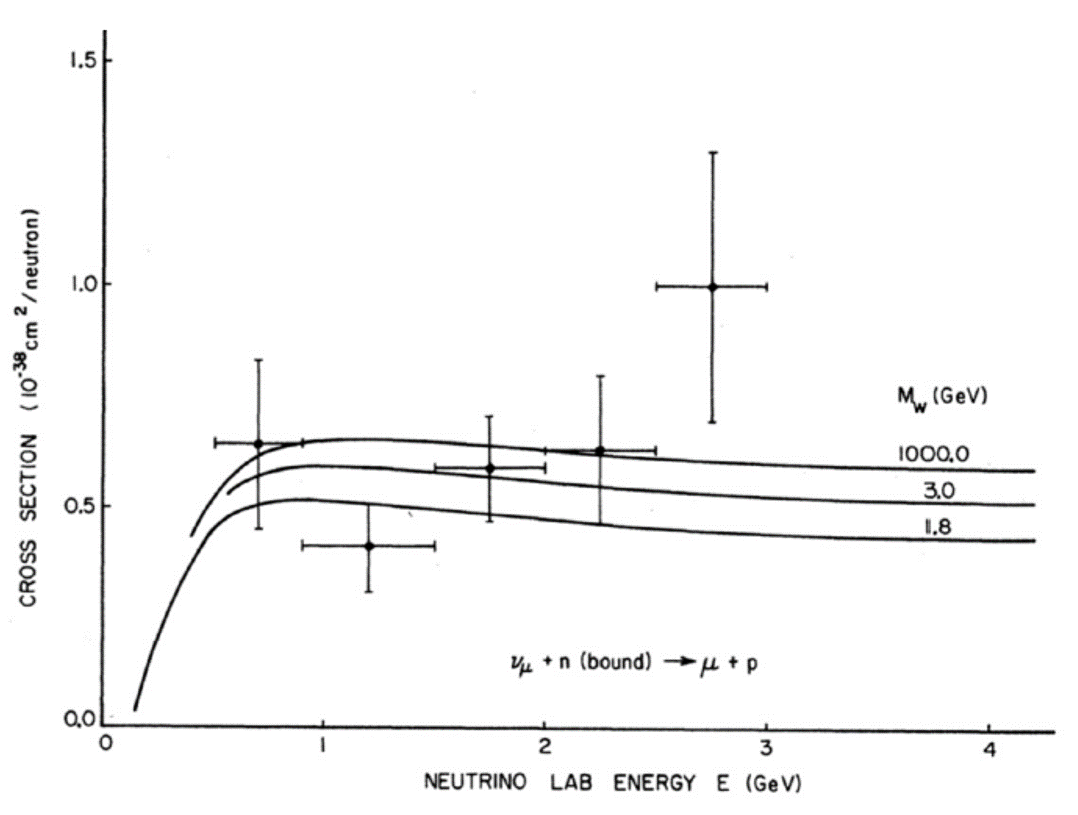## 1971

#### Neutrino-nucleon cross-section [publication]

Neutrino-nucleon cross-section measurement (the first one?)

Those points are experimental measurements of the cross section (probability of interaction) of nu_mu neutrinos on neutrons within nucleus of carbon atoms. They did not show yet significantly the increase of the cross-section with energy but confirmed the very low value predicted by theory (the 3 curves for the 3 different values of the W boson which was not yet known in 1971).

Plot to be added

## 1968

#### First Homestake results

The Homestake Chlorine experiment made several measurements of the number of solar neutrino interactions between 1964 and 1968. The first results that were compared to J. Bahcall prediction and showed, with large error bars, that the number of detected neutrinos were only 1/3 of the theoretical prediction.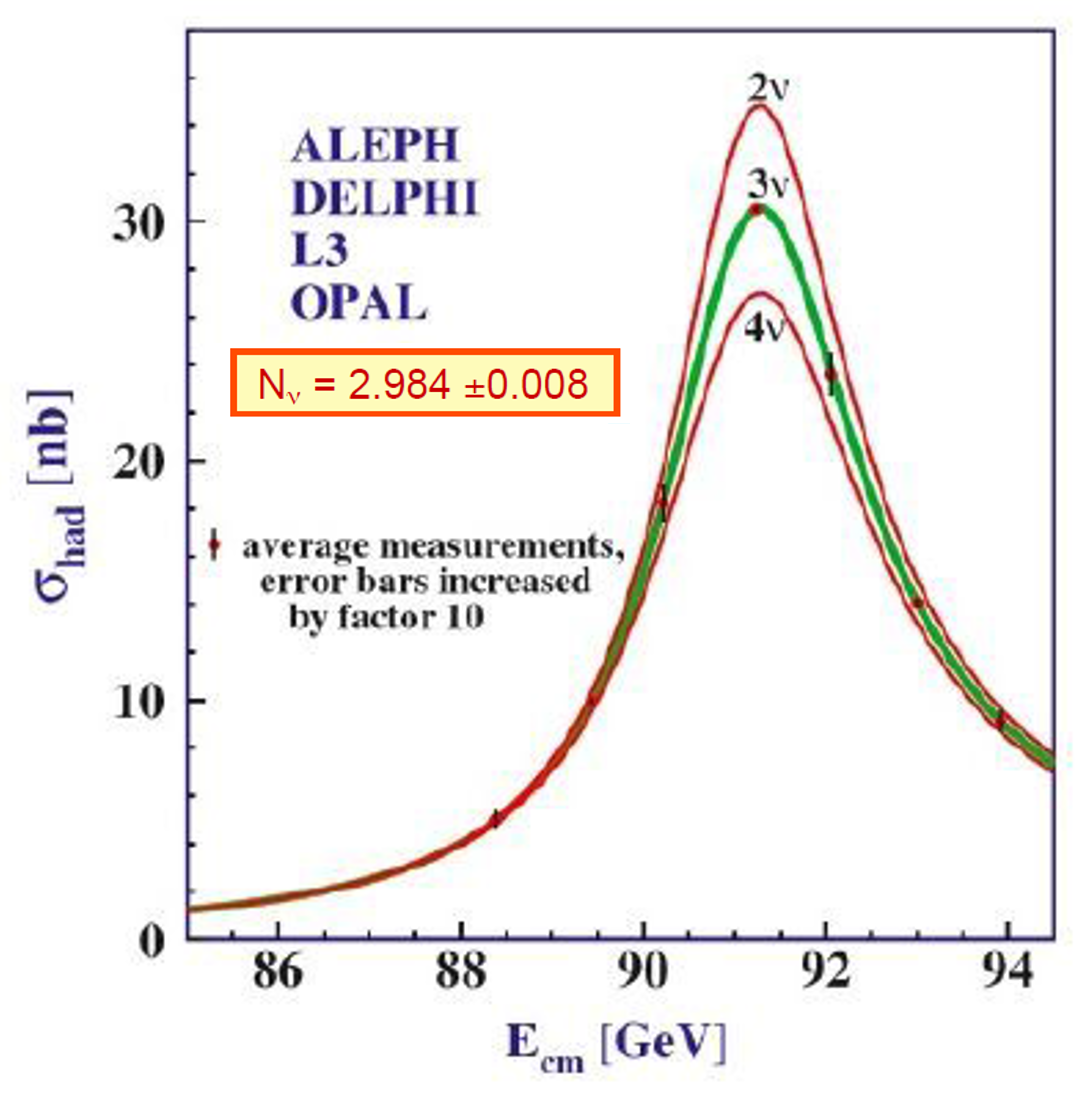## 1993

#### LEP finds 3 neutrino families

LEP measurements of the Z width and the resulting number of neutrino families

The measurement’s points are average hadronic cross-sections computed from the results of the 4 LEP experiments and showing the resonance corresponding to the Z boson. The colored curves are the theoretical predictions depending on the number of neutrinos types which are active in the weak interaction. The curve with 3 neutrino types best fits the measurements.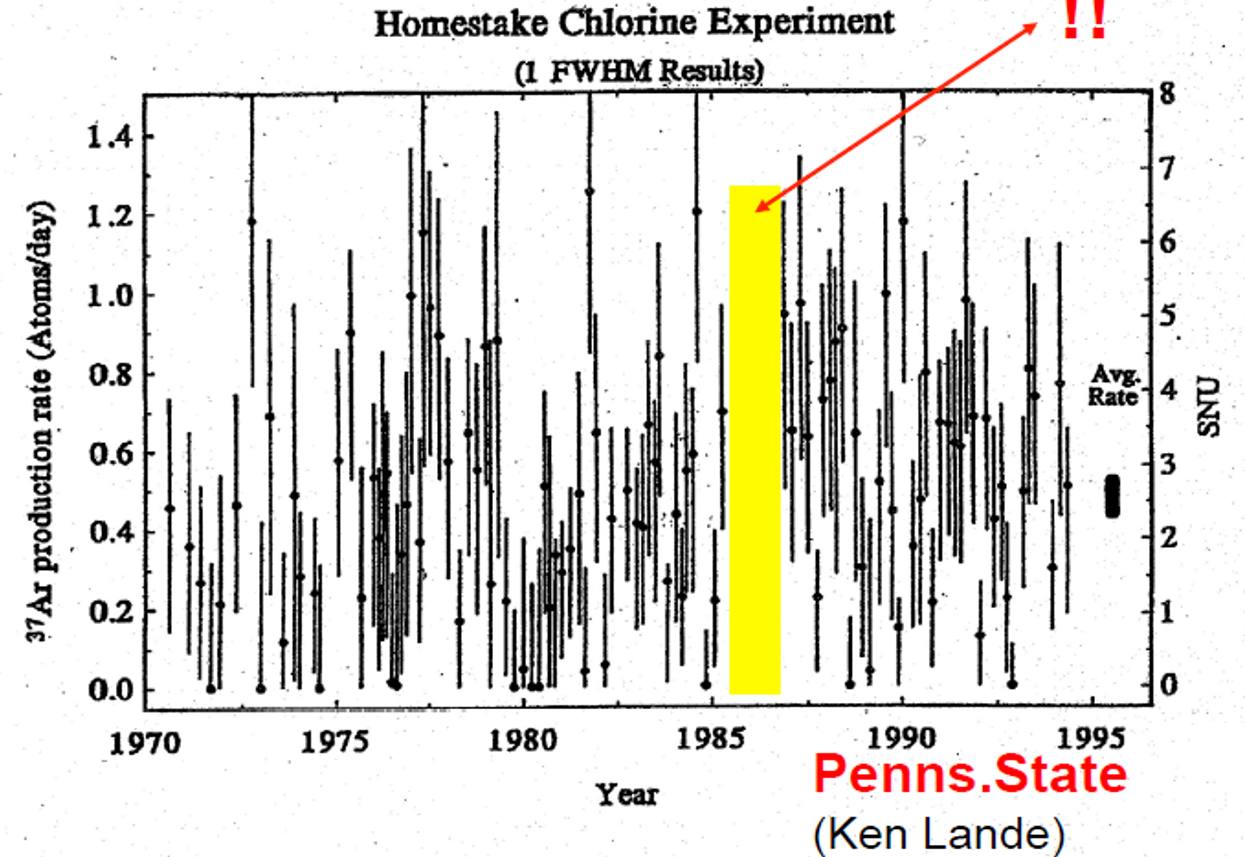## 1994

#### Homestake solar neutrinos summary

Homestake Chlorine solar neutrino results (1970-1994)

Each point is a daily rate of the number of radioactive Argon atoms detected by the radiochemical system which periodically scanned the 600 tons of chlorin solution of the detector. Each Argon atom was produced by a neutrino interaction with a Chlorin atom.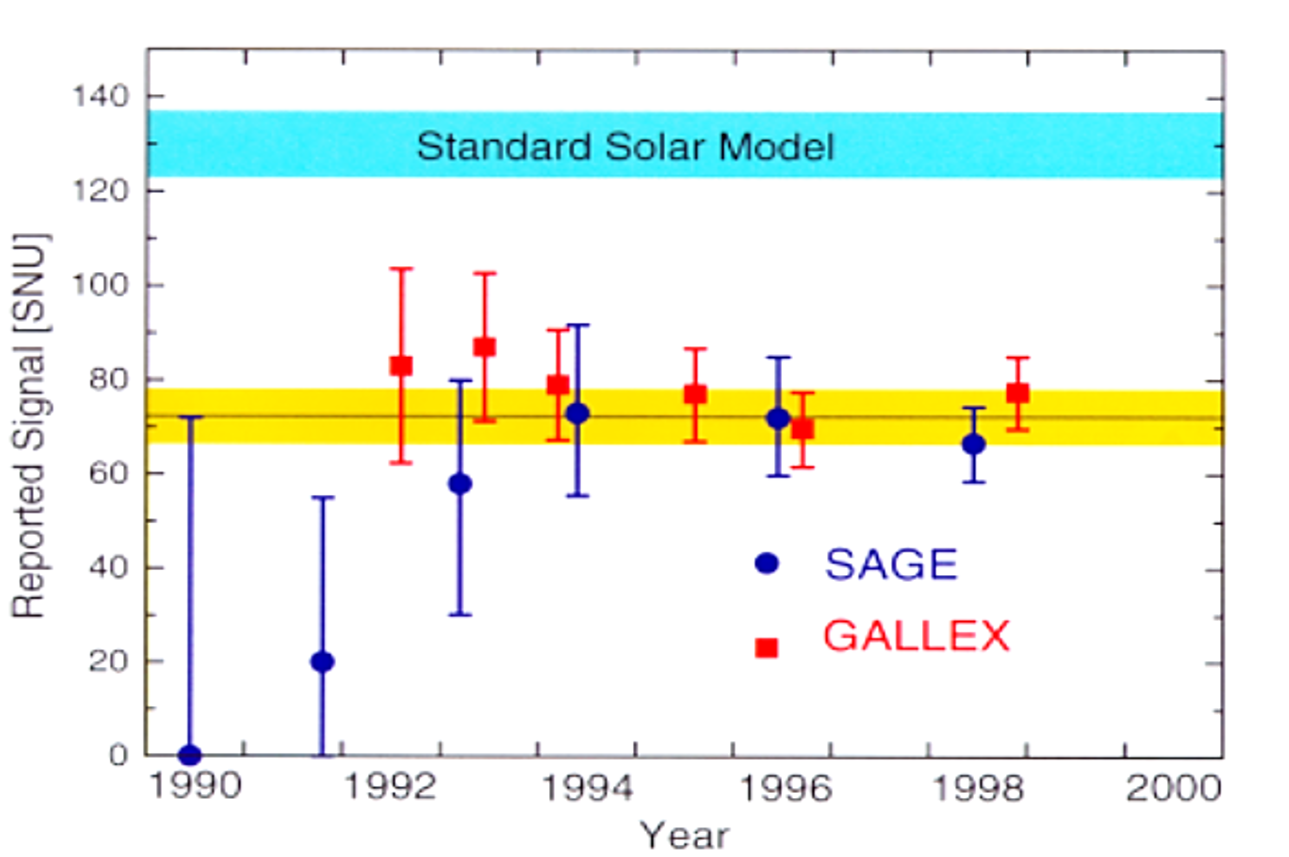## 1998

#### SAGE and GALLEX summary

Gallex and Sage experiments solar neutrino results (1990-1998)

Each point is the rate of Gallium atom trasnformed into Germanium by neutrino interaction and detected in SAGE or GALLEX experiments. This rate was measured approximately once per year and is shown here in SNU (Solar Neutrino Unit) which is the solar neutrino flux producing 10−36 captures per target atom per second.## 1998

#### SuperK first observation of neutrino oscillation

Neutrino oscillation result from SuperKamiokande

The SuperK experiment was able to measure for each neutrino detected the energy E and the length of fly of the neutrino. The number of neutrinos detected, normalized by the predicted number should have been always 1 if the neutrino oscillation does not occured. The red curve shows the theoretical prediction which best fit the measurement’s points.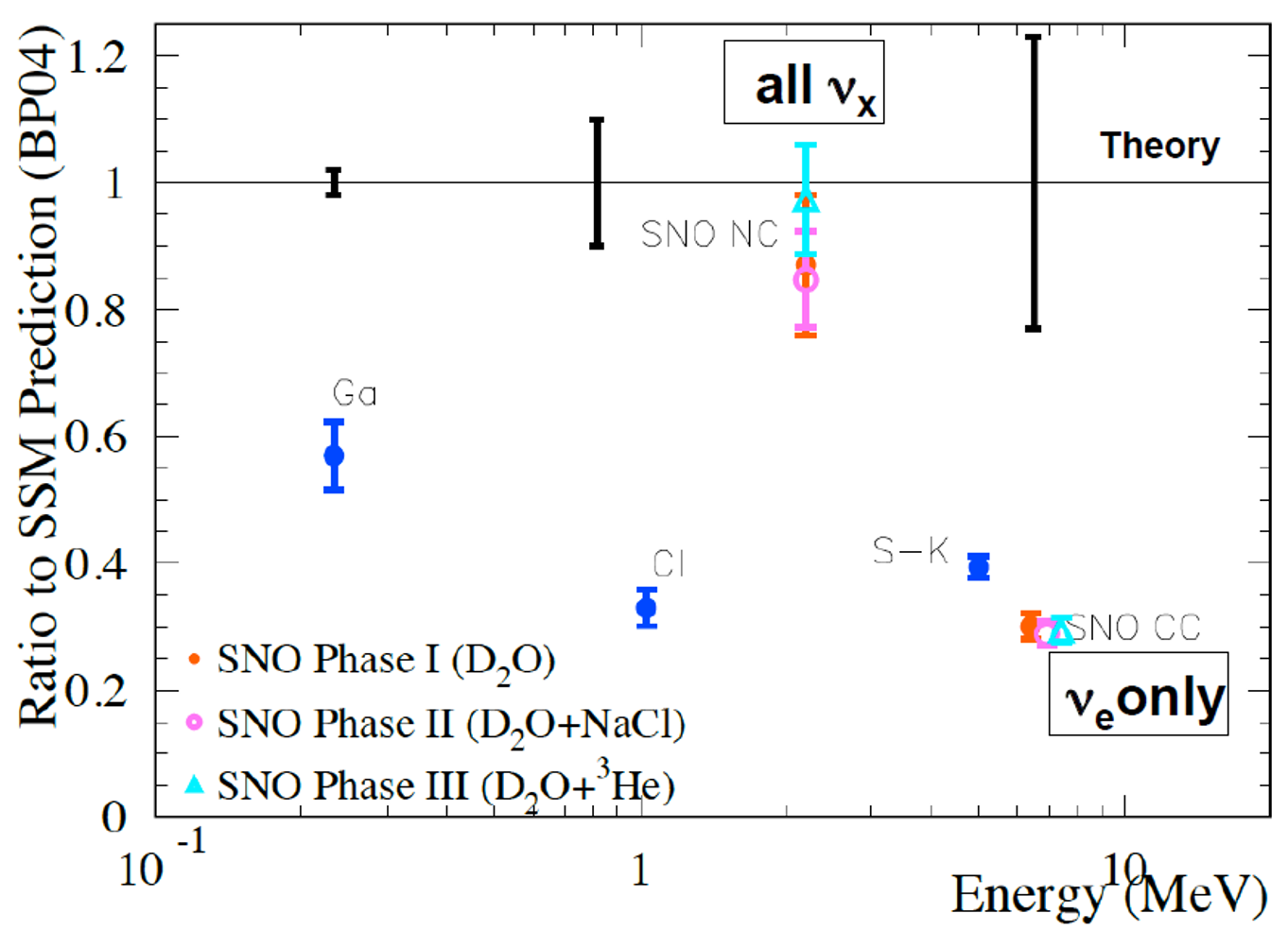## 2001

#### Solar neutrinos summary

SNO and other solar neutrino experiments results

Each point is a summary result of the various solar neutrino experiments (Gallex, Homestake, Super-Kamiokande and SNO) and shows the ratio between the measured neutrino flux and the predicted neutrino flux if there is no neutrino oscillation. The SNO NC (Neutral Current) measurement is compatible with the value 1 since all the neutrino flavors are detected in this case. Each experiment has a different result because it looks at solar neutrino of different energies.## 2002

#### KamLAND observes neutrino oscillation

KamLAND (and previous reactor experiments) neutrino oscillation result

Each point on this plot shows the number of neutrinos observed with respect to the number of neutrino expected if there were no neutrino oscillation. All the experiments before KamLAND were too close to the nuclear reactor to see the oscillation. The dashed curve shows the theoretical oscillation with the best fitting parameters for the KamLAND measurement’s point. The green area corresponds to the uncertainty on those parameters.## 2004

#### KamLAND sees full neutrino oscillation

Neutrino oscillation seen by KamLAND

The “Survival Probability” is computed from the number of electron anti-neutrinos produced by the nuclear reactor and detected by KamLAND. It depends on the ratio between the distance  KamLAND-nuclear reactor and the energy of the neutrinos. The measurement points shows clearly the oscillation phenomena and its dependence on L/E.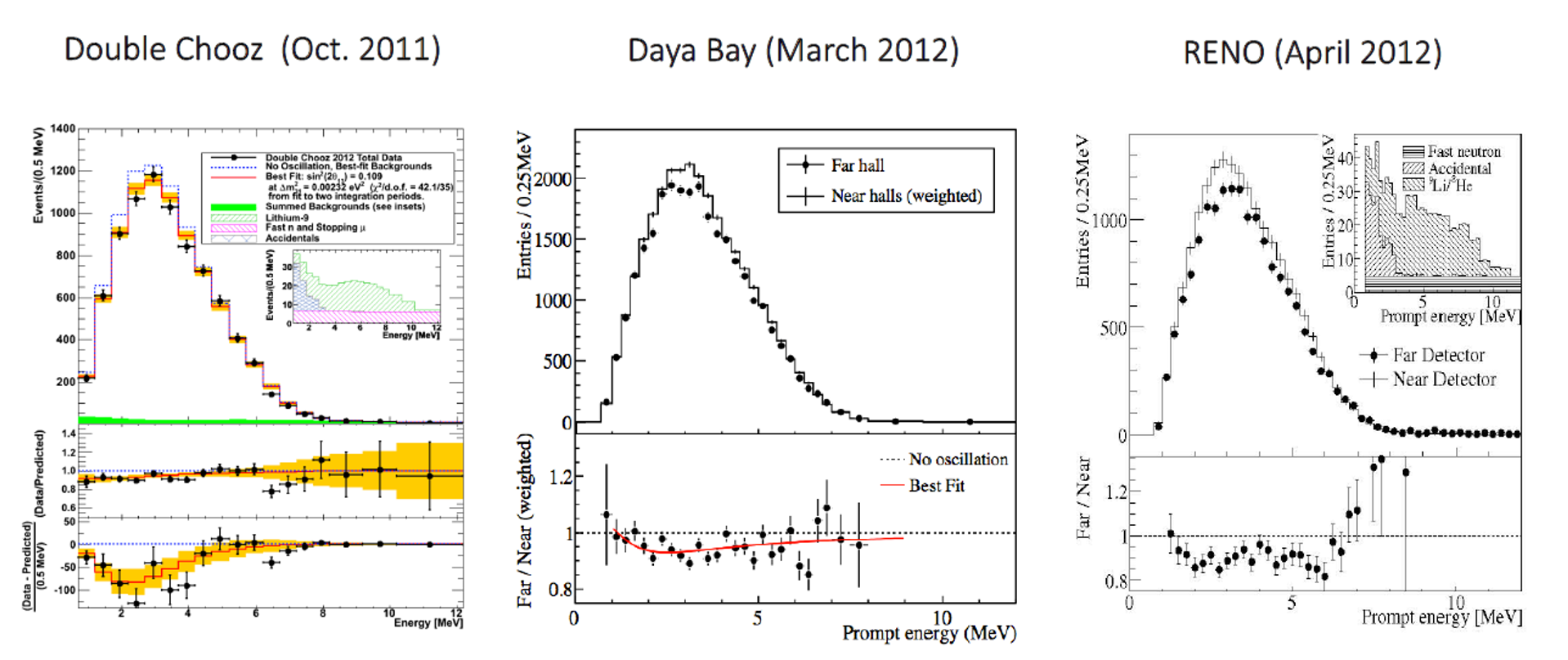## 2011

#### Last reactor neutrino results

Double Chooz, DayaBay and RENO reactor neutrino results

For each of those three experiments, the measreuement point of the upper plot are the number of neutrinos detected as a function of their energy, while the lower plots visualize the ratio between the measurements and the theoretical expectation in the case of no neutrino oscillation (Double Chooz) or the ratio between the number of neutrinos detected in the far and near detectors (Daya Bay, RENO), the near detector being the reference where no neutrino oscillation has yet occured.## 2013

#### Planck observes 3 neutrino families

Planck CMB measurement and the resulting number of neutrino families

The Planck satellite has measured the temperature of the Cosmic Microwave Background in all the directions of the Universe and looked at the temperature fluctuations for various angular scales (the l parameter on the X axis of this plot). The first peak around l=100 corresponds to large fluctuations linked to baryogenesis and is called the baryonic acoustic peak. The red curve is a fit to the data, of which one parameter is the number of neutrino families. The best fit gives 3 neutrino families with an uncertainty around 0.2.